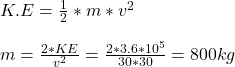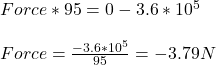## A car moves at a constant velocity of 30 m/s and has 3.6 × 105 J of kinetic energy. The driver applies the brakes and the car stops in 95 m.

Question

A car moves at a constant velocity of 30 m/s and has 3.6 × 105 J of kinetic energy. The driver applies the brakes and the car stops in 95 m.
Calculate the force needed to stop the vehicle.

in progress 0
5 months 2021-08-15T00:46:38+00:00 1 Answers 13 views 0

1. The force needed to the stop the car is -3.79 N.

Explanation:

The force required to stop the car should have equal magnitude as the force required to move the car but in opposite direction. This is in accordance with the Newton’s third law of motion. Since, in the present problem, we know the kinetic energy and velocity of the moving car, we can determine the mass of the car from these two parameters.

So, here v = 30 m/s and k.E. = 3.6 × 10⁵ J, then mass will beNow, we know that the work done by the brake to stop the car will be equal to the product of force to stop the car with the distance travelled by the car on applying the brake.Here it is said that the car travels 95 m after the brake has been applied. So with the help of work energy theorem,

Work done = Final kinetic energy – Initial kinetic energy

Work done = Force × Displacement

So, Force × Displacement = Final kinetic energy – Initial Kinetic energy.Thus, the force needed to the stop the car is -3.79 N.